# Colleges with the lowest SAT scores in New York

Top 10 colleges in New York with the lowest SAT scores
Looking for the colleges with the lowest SAT scores in New York? Well you're in luck! We've compiled a national college database and have created a list of the top 10 universities with the lowest SAT scores in New York below. If you are not a good test taker or worried about your test scores, this list is for you. These are the schools whose applicants had the lowest average SAT scores in New York, which means that you can get into these colleges with a lower SAT score. We also include each college's ACT scores and acceptance rate so that you can see where you would have the easiest time getting in. Read on to find out more.

## Morrisville State College SAT scores

The average SAT score for Morrisville State College is 945.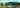The average SAT score of 945 breaks down into:

• SAT math: 470

The average ACT score for Morrisville State College is 19 and their acceptance rate is 71.9%.

## SUNY Cobleskill SAT scores

The average SAT score for SUNY Cobleskill is 970.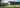The average SAT score of 970 breaks down into:

• SAT math: 480

The average ACT score for SUNY Cobleskill is 20 and their acceptance rate is 94%.

## Five Towns College SAT scores

The average SAT score for Five Towns College is 970.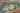The average SAT score of 970 breaks down into:

• SAT math: 480

The average ACT score for Five Towns College is 18 and their acceptance rate is 62.3%.

## The College of New Rochelle SAT scores

The average SAT score for The College of New Rochelle is 989.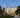The average SAT score of 989 breaks down into:

• SAT math: 470

The average ACT score for The College of New Rochelle is 22 and their acceptance rate is 68.4%.

## Nyack College SAT scores

The average SAT score for Nyack College is 990.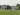The average SAT score of 990 breaks down into:

• SAT math: 490

The average ACT score for Nyack College is 20 and their acceptance rate is 98.4%.

## Canton State University of New York SAT scores

The average SAT score for Canton State University of New York is 990.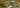The average SAT score of 990 breaks down into:

• SAT math: 495

The average ACT score for Canton State University of New York is 19 and their acceptance rate is 78%.

## Cazenovia College SAT scores

The average SAT score for Cazenovia College is 1010.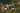The average SAT score of 1010 breaks down into:

• SAT math: 500

The average ACT score for Cazenovia College is 22 and their acceptance rate is 92.4%.

## Hartwick College SAT scores

The average SAT score for Hartwick College is 1010.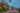The average SAT score of 1010 breaks down into:

• SAT math: 510

The average ACT score for Hartwick College is 24 and their acceptance rate is 88.6%.

## Dominican College SAT scores

The average SAT score for Dominican College is 1040.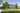The average SAT score of 1040 breaks down into:

• SAT math: 520

The average ACT score for Dominican College is 20 and their acceptance rate is 76.7%.

## Keuka College SAT scores

The average SAT score for Keuka College is 1040.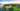The average SAT score of 1040 breaks down into:

• SAT math: 520